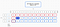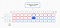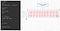# Dynamic Programming: Bottom-Up

Previously I talked about using recursion and memoization top-down approach to solving recursive problems(specifically the Fibonacci Sequence). This week I want to go over dynamic programming using an iterative bottom-up approach.

In the Fibonacci sequence a number in the sequence is equal to the previous two numbers in the sequence. n = (n-1) + (n- 2)

The recursive way would solve f(n) = f(n-1 ) + f(n -2) over and over even it it’s solved f(n). In the memoization way, it would check if f(n) was already solved and use that answer. In the bottom up approach, we will solve f(0) (the bottom) to f(n) iteratively.

First we’ll create an array (dp) based on the size of n. If we are trying to solve fib(6) dp = Array.new(n+1, 0) will create an array of [0,0,0,0,0,0,0].

We will also declare our base case. We know that the 0 and 1st numbers in Fibonacci will always be 0 and 1 respectively.

We then move onto our for loop. Since we already know our base case of 0 and 1, we’ll start our loop at 2 until we hit the end.

When i = 2. dp = dp[i -1] + dp[i -2] (dp + dp).

0 + 1 = 1 (dp = 1)

dp = dp + dp which is 2.

We’re just summing the previous 2 values (dp[i-1] + dp[i2])

We’ll keep doing this until i reaches 6.

When we return the function, we return dp[n] which in this case is dp. The 6th position of the Fibonacci sequence is 8.

When using a dp table, we want the last item in the table, which in this case is 8.

## A more complex case for using a DP Bottom-Up approach

A popular code challenge question that pops up is the coin change problem.

Given a value (n), and an array of coins of different values (k), how many possible ways are there to make N.

`exampleN = 10 (our target value)K = [1, 5, 10] (our array of coins)There are 4 different ways to make 10 cents.`

## Our DP table

when K is 0 our options for coins are 0 (none) and 1. When the target is 0 using 0 coins we can have 1 possible way of making 0 (0 coins). When the target is 1, there is 1 possible way using 1 1cent coin. When the target is 2, there is 1 possible way using 1 + 1 cent coins. This goes all the way to 10.

So if we have an array of [[0,0,0],[0,0,0],[0,0,0],[0,0,0],[0,0,0],[0,0,0],[0,0,0],[0,0,0],[0,0,0],[0,0,0],[0,0,0]]. We know that index 0, is 1 for every since one.

[[1,0,0],[1,0,0],[1,0,0],[1,0,0],[1,0,0],[1,0,0],[1,0,0],[1,0,0],[1,0,0],[1,0,0],[1,0,0]]

We can also say, that using 0 coins, we can always have 1 way to make 0.

`our base case would be[[1,1,1],[1,0,0],[1,0,0],[1,0,0],[1,0,0],[1,0,0],[1,0,0],[1,0,0],[1,0,0],[1,0,0],[1,0,0]]`

Now, lets look at 5. Using a set of coins, 0,1, and 5. We can’t make a target of 1 using 5. 5 doesn’t going into 1.red boxes are solved. solid blue box is the 5 into 1, which is 0.

But, we know that in a set of 1 and 5, there is 1 possible way to reach a target of 1. this is represented as k-1 (for now)

So, dp[n][k] is equal to dp[n][k] + dp[n][k-1]. Using that logic, we can at least fill in the table to dp.

When we reach dp, k(5) goes into n(5) once! So we can say, we can hit the target of 5 using 1 5 cent coin. We also know, we can a target of 5 using 5 1 cent coins. There’s 2 possible ways.

`dp[n][k] = (dp[n] / dp[k]) + dp[n][k-1]`using the coin itself(dark blue) and using the previous coins possible solutions

So, 1 + 1 = 2

When we reach n = 10, k(5) goes into 10 twice. plus the previous solution for 1, we get 2 + 1 = 3

Using the formula, dp[n][k] = (dp[n] / dp[k]) + dp[n][k-1], the bottom row excluding the last box would look like the following.

We can see 10 goes into 10 once. Adding dp[n][k-1](3) we get 4.

Our expected output should be 4.

## Putting it together

First we need to setup our array. Our array should be the length of n + 1 (dont forget arrays start at 0 and we need index of n)

If you recall our base case, every dp[n] is 1 and k for dp[k] is 1, we can set this up by doing the following.

## Now, onto the next part

We want to iterate through every target of n to figure out how many possible combinations of coins (k) to reach n.

The formula we came up with was dp[n][k] = (dp[n] / dp[k]) + dp[n][k-1]

Using our i and j loops we can write this as:

`dp[i][j] = (i / k[j]) + dp[i][j-1]since i represents n and k[j] represents the current coin`

If we compare output to our dp table, we can see it matches up.

Since we want to return the last box(4) (the 4 possible ways to get N using K coins) We need to return the last index of the last inner array. We can do this by using

`return dp[-1][-1] # -1 get the last item of the array. # dp[-1] is [1,3,4]# dp[-1][-1] is 4`using dp[-1][-1] we can target the last “box” which is 4

Bottom up can be a bit confusing and hard to wrap your ahead around it, but with more practice you’ll get used to it.

Fullstack Software Engineer

## More from Alex Duterte

Fullstack Software Engineer